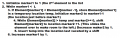# Comparisons in Insertion Sort

#### zulfi100

Joined Jun 7, 2012
656
Hi,
Let us look at the amount of work that is required to insert the last element into its proper place. If the list length is L, we expect about L/2 comparisons & L/2 assignments
I cant understand the above statement. I have attached an example & by looking at that i cant understand how we have L/2 comparisons

The algorithm for Insertion sort is:Some body please guide me with this comparison problem.

Zulfi.

#### WBahn

Joined Mar 31, 2012
27,866
First, let's use the variable S to represent the number of values in the list that have to be shifted in order to insert the last element into its proper place.

In other words, consider the following list in which we want to insert the last element into its proper place.

1 2 4 5 6 7 8 9 3

S, in this case, would be 6

Figure out how many comparisons and assignments are required to move the 3 into its final position in terms of S.

The only remaining question is what the value of S is, on average. Sometimes it will be 0 (though you will always have at least one comparison to make) and sometimes it will be L (the entire list has to be shifted). But, on average, what do you expect it to be? This is assuming that the list being sorted is effectively random.

#### WBahn

Joined Mar 31, 2012
27,866
Oh, and surely you could get that monster attachment down to a much more reasonable size, couldn't you?

•absf

#### zulfi100

Joined Jun 7, 2012
656
Hi,

Thanks for your reply. I have got some confusion. S represents number of shifts. To insert 3 at its proper place there would be 6 shifts (fine, as you say). But comparisons would be more. If you look at the algorithm, loop starts at line#2. But comparisons are starting from line#3. So first comparison would be:

2 < 1 (false: no shifting) then

4 < 2 (false: no sifting) then

5 < 4 (false: no shifting) then

6 < 5 (false: no shifting) then

7 < 6 (false: no shifting) then

8 < 7 (false: no shifting) then

9 < 8 (false: no shifting) then

3 < 9 (true: first shifting occurs & marker2 comes into play)

Now we will execute the loop starting at line#4. There would be shifting i.e 6 & again comparisons probably 6, so altogether 13 comparisons. Am I right, please guide me?

Zulfi.

#### WBahn

Joined Mar 31, 2012
27,866
Look at the algorithm more closely. You start at the other end.

0 1 2 3 4 5 6 7 8 (index)
1 2 4 5 6 7 8 9 3 (value)

v < v ? = True => temp = 3, v = v
v < temp ? = True => v = v
v < temp ? = True => v = v
v < temp ? = True => v = v
v < temp ? = True => v = v
v < temp ? = True => v = v
v < temp? = False => v = temp

Remember, the question is asking about the number of operations needed to move the last element in the list (which is the item that Marker1 is currently pointing to) into its proper location. That means that the algorithm has already been run long enough to make it so that everything but the last element is already sorted.

#### zulfi100

Joined Jun 7, 2012
656
Hi,
0 1 2 3 4 5 6 7 8 (index)
1 2 4 5 6 7 8 9 3 (value)

v < v ? = True => temp = 3, v = vc

Sorry my teacher:
v = 9
v = 3
9<3?= False???

Any way I accept that there would be 6 comparison. But on the average how it can be L/2??

Zulfi.

#### WBahn

Joined Mar 31, 2012
27,866
Consider all of the possible cases.

How many comparisons will there be if the final value is already in its final position?

How many will there be when it only has to be moved back one place? Two places? Three? All but one? All the way to the first?

What's the average?

#### zulfi100

Joined Jun 7, 2012
656
final pos = 1
one place = 2
two place = 3
three place = 4
four place = 5
five place = 6
six place = 7
seven place = 8
eight place = 9
nine place = 10
avg = 55/9 = 6.11
where as n/2 = 4.5

So what's wrong here?

Zulfi.

#### WBahn

Joined Mar 31, 2012
27,866
On thing that you are forgetting is that these analyses are asymptotic for large values of n, so you are looking at what they converge to, not what their exact values are.